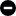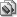# BugID: #00161

カテゴリ 重要度 ステータス 解決状況 登録日時 最終更新クラッシュ/フリーズ重要 (本体)解決済み修正済み2008-01-25 02:432008-01-25 04:12

テスターFirewave担当者 ソースscregg.cpp
バージョン0.115発生バージョン修正バージョン0.115u1
フラグ旧BugIDscregg0115red
セット scregg, eggs, dommy, rockduck
セット詳細dommy - ドミーeggs - スクランブルエッグ / Eggs (US版)rockduck - Rock Duck (プロトタイプ?)scregg - スクランブルエッグ

This first happens in 0.114u4. There were some 6502 cpu changes in that U release which might be casuing the issue. The driver itself has not been touched in nearly 3 months.

```(gdb) run scregg -window

Program received signal SIGSEGV, Segmentation fault.
0x00e8f4f8 in videoram_w (offset=0, data=0 '\0') at src/emu/video/generic.c:390
390             dirtybuffer[offset] = 1;
(gdb) bt full
#0  0x00e8f4f8 in videoram_w (offset=0, data=0 '\0')
at src/emu/video/generic.c:390
data = 0 '\0'
#1  0x00ea347b in program_write_byte_8 (address=0, data=0 '\0')
at src/emu/memory.c:2723
entry = 80
data = 0 '\0'
#2  0x01290029 in m6502_91 () at src/emu/cpu/m6502/t6502.c:67
tmp = 0
#3  0x012a6ef7 in m6502_execute (cycles=1644) at src/emu/cpu/m6502/m6502.c:235
op = 145 '\221'
#4  0x00eb2340 in cpunum_execute (cpunum=0, cycles=1644)
at src/emu/cpuintrf.c:1389
ran = 0
#5  0x00ef9d16 in cpuexec_timeslice () at src/emu/cpuexec.c:502
target = {seconds = 0, subseconds = 6796421052631575}
base = {seconds = 0, subseconds = 5699929824561400}
cpunum = 0
ran = 1643
#6  0x00e91219 in run_game (driver=0x14fadf0) at src/emu/mame.c:346
machine = (running_machine *) 0x4077360
error = 0
firstrun = 0
mame = (mame_private *) 0x40776a0
cb = (callback_item *) 0x21d044e
#7  0x00f1d4d0 in cli_execute (argc=3, argv=0x3fa25c0, osd_options=0x214d3a0)
at src/emu/clifront.c:268
exename = 0x3fa2680 "mame115symbols"
sourcename = 0x4075858 "scregg"
gamename = 0x3faffd8 "scregg"
driver = (const game_driver *) 0x14fadf0
drv = {driver_data_size = 0, cpu = {{cpu_type = 5, cpu_flags = 0,
cpu_clock = 1500000, construct_map = {{
0xc5bfc6 ,
0xc5c249 }, {0, 0}, {0, 0}},
vblank_interrupt = 0xe9d7f1 ,
vblank_interrupts_per_frame = 16, timed_interrupt = 0,
timed_interrupt_period = 0, reset_param = 0x0, tag = 0x0}, {
cpu_type = 0, cpu_flags = 0, cpu_clock = 0, construct_map = {{0, 0}, {0,
0}, {0, 0}}, vblank_interrupt = 0, vblank_interrupts_per_frame = 0,
timed_interrupt = 0, timed_interrupt_period = 0, reset_param = 0x0,
tag = 0x0}, {cpu_type = 0, cpu_flags = 0, cpu_clock = 0,
construct_map = {{0, 0}, {0, 0}, {0, 0}}, vblank_interrupt = 0,
vblank_interrupts_per_frame = 0, timed_interrupt = 0,
timed_interrupt_period = 0, reset_param = 0x0, tag = 0x0}, {
cpu_type = 0, cpu_flags = 0, cpu_clock = 0, construct_map = {{0, 0}, {0,
0}, {0, 0}}, vblank_interrupt = 0, vblank_interrupts_per_frame = 0,
timed_interrupt = 0, timed_interrupt_period = 0, reset_param = 0x0,
tag = 0x0}, {cpu_type = 0, cpu_flags = 0, cpu_clock = 0,
construct_map = {{0, 0}, {0, 0}, {0, 0}}, vblank_interrupt = 0,
vblank_interrupts_per_frame = 0, timed_interrupt = 0,
timed_interrupt_period = 0, reset_param = 0x0, tag = 0x0}, {
cpu_type = 0, cpu_flags = 0, cpu_clock = 0, construct_map = {{0, 0}, {0,
0}, {0, 0}}, vblank_interrupt = 0, vblank_interrupts_per_frame = 0,
timed_interrupt = 0, timed_interrupt_period = 0, reset_param = 0x0,
tag = 0x0}, {cpu_type = 0, cpu_flags = 0, cpu_clock = 0,
construct_map = {{0, 0}, {0, 0}, {0, 0}}, vblank_interrupt = 0,
vblank_interrupts_per_frame = 0, timed_interrupt = 0,
timed_interrupt_period = 0, reset_param = 0x0, tag = 0x0}, {
cpu_type = 0, cpu_flags = 0, cpu_clock = 0, construct_map = {{0, 0}, {0,
0}, {0, 0}}, vblank_interrupt = 0, vblank_interrupts_per_frame = 0,
timed_interrupt = 0, timed_interrupt_period = 0, reset_param = 0x0,
tag = 0x0}}, cpu_slices_per_frame = 0, watchdog_vblank_count = 0,
watchdog_time = 0, machine_start = 0, machine_reset = 0, nvram_handler = 0,
memcard_handler = 0, video_attributes = 0, gfxdecodeinfo = 0x1fca190,
total_colors = 8, color_table_len = 8, default_layout = 0x0, screen = {{
tag = 0x2152f50 "main", palette_base = 0, defstate = {width = 256,
height = 256, visarea = {min_x = 8, max_x = 247, min_y = 8,
max_y = 247}, oldstyle_vblank_supplied = 1 '\001',
refresh = 17543859649122807, vblank = 3072000000000000,
format = BITMAP_FORMAT_INDEXED16}}, {tag = 0x0, palette_base = 0,
defstate = {width = 0, height = 0, visarea = {min_x = 0, max_x = 0,
min_y = 0, max_y = 0}, oldstyle_vblank_supplied = 0 '\0',
refresh = 0, vblank = 0, format = BITMAP_FORMAT_INVALID}}, {tag = 0x0,
palette_base = 0, defstate = {width = 0, height = 0, visarea = {
min_x = 0, max_x = 0, min_y = 0, max_y = 0},
oldstyle_vblank_supplied = 0 '\0', refresh = 0, vblank = 0,
format = BITMAP_FORMAT_INVALID}}, {tag = 0x0, palette_base = 0,
defstate = {width = 0, height = 0, visarea = {min_x = 0, max_x = 0,
min_y = 0, max_y = 0}, oldstyle_vblank_supplied = 0 '\0',
refresh = 0, vblank = 0, format = BITMAP_FORMAT_INVALID}}, {tag = 0x0,
palette_base = 0, defstate = {width = 0, height = 0, visarea = {
min_x = 0, max_x = 0, min_y = 0, max_y = 0},
oldstyle_vblank_supplied = 0 '\0', refresh = 0, vblank = 0,
format = BITMAP_FORMAT_INVALID}}, {tag = 0x0, palette_base = 0,
defstate = {width = 0, height = 0, visarea = {min_x = 0, max_x = 0,
min_y = 0, max_y = 0}, oldstyle_vblank_supplied = 0 '\0',
refresh = 0, vblank = 0, format = BITMAP_FORMAT_INVALID}}, {tag = 0x0,
palette_base = 0, defstate = {width = 0, height = 0, visarea = {
min_x = 0, max_x = 0, min_y = 0, max_y = 0},
oldstyle_vblank_supplied = 0 '\0', refresh = 0, vblank = 0,
format = BITMAP_FORMAT_INVALID}}, {tag = 0x0, palette_base = 0,
defstate = {width = 0, height = 0, visarea = {min_x = 0, max_x = 0,
min_y = 0, max_y = 0}, oldstyle_vblank_supplied = 0 '\0',
refresh = 0, vblank = 0, format = BITMAP_FORMAT_INVALID}}},
init_palette = 0x576ea0 ,
video_start = 0x5771d2 , video_reset = 0, video_eof = 0,
video_update = 0x577bf9 , sound = {{sound_type = 6,
clock = 1500000, config = 0x0, tag = 0x0, routes = 1, route = {{
output = -1, target = 0x1fca1c0 "mono", gain = 0.230000004}, {
output = 0, target = 0x0, gain = 0} }}, {
sound_type = 6, clock = 1500000, config = 0x0, tag = 0x0, routes = 1,
route = {{output = -1, target = 0x1fca1c0 "mono", gain = 0.230000004}, {
output = 0, target = 0x0, gain = 0} }}, {
sound_type = 0, clock = 0, config = 0x0, tag = 0x0, routes = 0, route = {
{output = 0, target = 0x0,
gain = 0} }} }, speaker = {{
tag = 0x1fca1c0 "mono", x = 0, y = 0, z = 1}, {tag = 0x0, x = 0, y = 0,
z = 0}, {tag = 0x0, x = 0, y = 0, z = 0}, {tag = 0x0, x = 0, y = 0,
z = 0}}, sound_start = 0, sound_reset = 0}
result = -1
#8  0x00e4e2a9 in utf8_main (argc=3, argv=0x3fa25c0)
at src/osd/windows/winmain.c:279
ext = 0x270855e ".map"
#9  0x0143a071 in main (argc=3, a_argv=0x3fa2500) at src/osd/windows/main.c:67
i = 3
rc = 58
utf8_argv = (char **) 0x3fa25c0
argv = (TCHAR **) 0x3fa2500
```